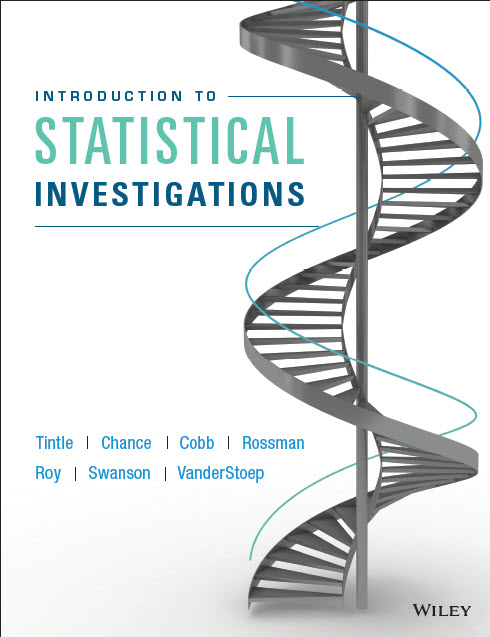Introduction to Statistical Investigations by Nathan Tintle, Beth Chance, George Cobb, Allan Rossman, Soma Roy, Todd Swanson, and Jill VanderStoep INFORMATIONAL VIDEOSThe following informational videos were prepared to give an idea of how we use randomization and simulation techniques in our introductory statistics curriculum. Introdution to Chance Models: Students are led through the first example in Chapter 1 (Can Dolphins Communicate?) as they discover the logic of inference using a single proportion. While building on their own intuition, they use coin flipping to model a null distribution and then speed up the process with the use of an applet. Chance Models: In this introduction to chance models we take a quick look at how we introduce inference during the first week of the semester. This is done by testing a single proportion. At this point, we do not use traditional statistics terminology like null hypothesis or p-value. That will come in the next section. Two Proportions: This video shows a simulation-based method in statistics that can be used to test two proportions. These tests are sometimes called randomization tests or permutation tests. Two Means: In this video we show how to do a simulation-based test for two means taken from independent samples. This test is quite similar to that of testing two proportions. Paired Data: A simulation-based method for testing paired data is shown in this video. Students at this point would have seen tests for a single proportion, single mean, two proportions, and two means from independent samples.